Next: Results Up: FINDING REARRANGEMENT PATHWAYS Previous: L-BFGS Minimiser   Contents

# A Single-ended Method: Eigenvector-following

Single-ended methods use only the function and its derivatives to search for a transition state from the starting point (the initial guess). Since a transition state is a local maximum in one direction but a minimum in all the others it is not generally possible to use standard minimisation methods for this purpose.

Newton-type optimisation methods are based on approximating the objective function locally by a quadratic model and then minimising that function approximately using, for example, the Newton-Raphson (NR) approach . However, the NR algorithm can converge to a stationary point of any index . To converge to a transition state the algorithm may need to start from a geometry at which the Hessian has exactly one negative eigenvalue and the corresponding eigenvector is at least roughly parallel to the reaction coordinate. Locating such a point can be a difficult task and several methods have been developed that help to increase the basins of attraction of transition states, which gives more freedom in choosing the starting geometry [9,22,24,19].

The most widely used single-ended transition state search method is eigenvector-following (EF). In it simplest form it requires diagonalisation of the Hessian matrix [12,14,13,15]. For larger systems it is advantageous to use hybrid EF methods that avoid either calculating the Hessian or diagonalising it [22,133,23,8].

We start by reviewing the theory behind the Newton-Raphson methods. Solving the eigenvalue problem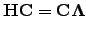(4.21)

yields the matrix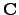, the columns of which are the eigenvectors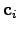, and the diagonal matrix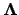, with eigenvalues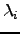on the diagonal. The matrixdefines a unitary transformation to a new orthogonal basis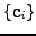, in which the original Hessian matrix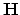is diagonal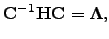(4.22)

so that Equation 2.13 is simplified to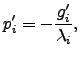(4.23)

where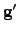is the gradient vector in the new basis, which is related to the original gradient vector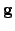as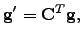(4.24)

because of the unitarity of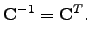(4.25)

The unnormalised search direction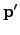is defined similarly.

After taking a step of length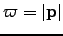in the direction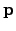, as prescribed by Equation 2.23, the energy changes by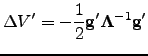(4.26)

provided our quadratic approximation to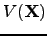is perfect. In general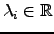and terms in Equation 2.26 with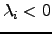and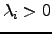increase and decrease the energy, respectively. For isolated molecules and bulk models with periodic boundary conditions the Hessian matrix is singular: there are three zero eigenvalues that correspond to overall translations. Non-linear isolated molecules have three additional zero eigenvalues due to rotations. Luckily, in all cases for which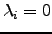the analytic form of the corresponding eigenvectorsis known so the eigenvalue shifting procedure could be applied: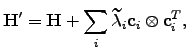(4.27)

where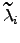are the new eigenvalues. In this work all the zero eigenvalues were shifted by the same amount (). As a result,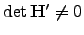and problems with applying Equation 2.23 do not arise.

The analytic forms of the's corresponding to translations along the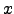,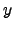and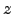directions are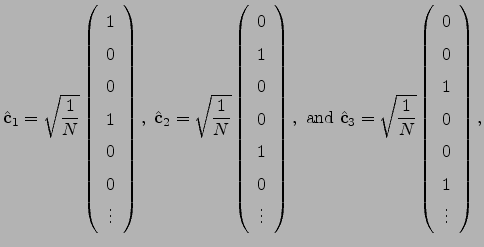(4.28)

respectively. The eigenvector that corresponds to rotation about theaxis by a small angle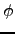can be obtained as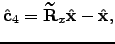(4.29)

where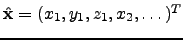is a normalised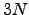-dimensional vector describing the position before the rotation, and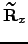is Maclaurin series expansion of the-dimensional rotation matrix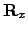with respect to a small angletruncated to the second order. The displacement vectors due to infinitesimal rotation about the,andaxes are therefore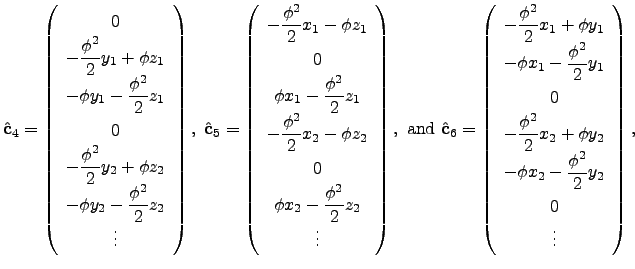(4.30)

respectively.

If started from a point at which the Hessian matrix has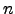negative eigenvalues, the NR method is expected to converge to a stationary point of index. However, the step taking procedure may change the index as optimisation proceeds.

Introducing Lagrange multipliers allows a stationary point of specified index to be located. Consider a Lagrangian function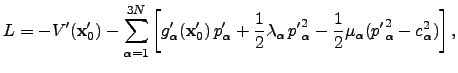(4.31)

where a separate Lagrange multiplier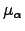is used for every eigendirection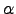,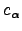is a constraint on the step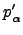,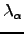is theth eigenvalue of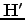, which is defined in Equation 2.27, and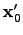is the point about which the potential energy function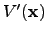was expanded. Primes, as before, denote that components are expressed in the orthogonal basis. Differentiating Equation 2.31 with respect toand using the condition for a stationary point the optimal step can be obtained: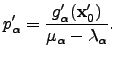(4.32)

The predicted energy change corresponding to this step is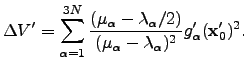(4.33)

We have some freedom in choosing the Lagrange multipliersas long as for the eigendirections for which an uphill (downhill) step is to be taken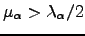(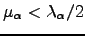). The following choice of Lagrange multipliers allows the recovery of the Newton-Raphson step in the vicinity of a stationary point :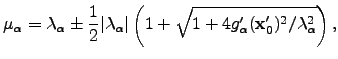(4.34)

with the plus sign for an uphill step, and the minus sign for a downhill step.

In cases when Hessian diagonalisation is impossible or undesirable the smallest eigenvalue and a corresponding eigenvector can be found using an iterative method .Next: Results Up: FINDING REARRANGEMENT PATHWAYS Previous: L-BFGS Minimiser   Contents
Semen A Trygubenko 2006-04-10